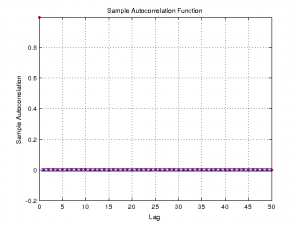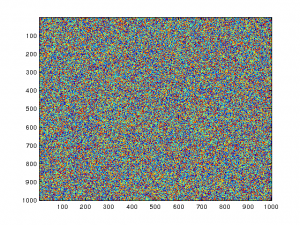## Using Arduino Due’s True Random Number Generator

Arduino Due uses an Atmel SAM3X8E ARM Cortex-M3 CPU, which has a native hardware based True Random Number Generator (TRNG). In this post, we will take a brief look at how to use it in the Arduino environment and take a look at some of its statistical characteristics.

The code below shows the easiest way to use the built-in TRNG:

```void setup() {
Serial.begin(9600);
pmc_enable_periph_clk(ID_TRNG);
trng_enable(TRNG);
}

void loop() {
Serial.println(t);
delay(10);
}
```

pmc_enable_periph_clk is defined in hardware/arduino/sam/system/libsam/source/pmc.c, and trng_enable, trng_read_output_data are both defined in hardware/arduino/system/libsam/source/trng.c. Technically speaking we need to wait till the random number generator is ready before reading the output data. But since the TRNG on SAM3X8E is fast (one random number for every 84 clock cycles), we can safely ignore it here. In the code above, each generated random number is treated as an unsigned 32 bit integer.

Alternatively, we can enable TRNG interrupt and use an interrupt handler to retrieve the generated random number when it is ready:

```void setup() {
Serial.begin(9600);
pmc_enable_periph_clk(ID_TRNG);
trng_enable(TRNG);

NVIC_DisableIRQ(TRNG_IRQn);
NVIC_ClearPendingIRQ(TRNG_IRQn);
NVIC_SetPriority(TRNG_IRQn, 0);
NVIC_EnableIRQ(TRNG_IRQn);
trng_enable_interrupt(TRNG);
}

void TRNG_Handler(void) {
uint32_t stat = trng_get_interrupt_status(TRNG);

if ((stat & TRNG_ISR_DATRDY) == TRNG_ISR_DATRDY) {
Serial.println(r);
}
}

void loop() {
}
```

Here we are checking the TRNG ISR status to ensure that the random number has been generated before reading the output data. Note that the result returned trng_read_output_data can be interpreted as either signed or unsigned numbers. In this particular example we are treating it as a signed 32 bit integer and thus the returned random number series will be distributed evenly around 0. Nested vectored interrupt controller NVIC_* routines are inlined in hardware/arduino/sam/system/CMSIS/CMSIS/Include/core_cm3.h.

The datasheet states that the TRNG in SAM3X8E passes the American NIST Special Publication 800-22 and Diehard Random Tests. Here we will take a look at some of the statistical characteristics of the pseudo random numbers generated via SAM3X8E. For the analysis below, I used the code listed above and generated 1 million samples.

The first figure shows the autocorrelation of the random number series when 1000 samples are used. The second figure shows the autocorrelation when 10,000 samples are used. As more samples are used, the autocorrelation of the sequence across different lags slowly converges to zero, indicating that the sequence is independently and identically distributed.

Here is the autocorrelation plot when the entire sample sequence (1 million) is used. As you can see, the autocorrelations are virtually zero across different lags.Here are two pictures showing the histogram and power spectrum density of the 1,000,000 samples. From these plots, we can infer how even these random numbers are distributed.

Here is another visual representation of the 1,000,000 sample data from the TRNG. The data is arranged into a 1000×1000 matrix and the color indicates the magnitude of the value at that point:1.Alexander says:

How is this any more ‘random’ than any other random number generator? I thought there was no true ‘random number generator’, that all use some algorithm with a seed? Even if you cycle the seed, you can still recreate the pattern?

•kwong says:

Well, by definition, a true random number generator should reply on some random physical phenomenon rather than just algorithms used in pseudorandom number generation. But I doubt that this chip is relying on an underlying physical process since the random number is generated every 84 clock cycles I am pretty sure it is still technically a pseudorandom number generator.

That said, some rngs are better than others and to pass the stringent die hard test, the statistical quality of the random numbers generated has to be very good.

2.David says:

You said: “As more samples are used, the autocorrelation of the sequence across different lags slowly converges to zero, indicating that the sequence is independently and identically distributed.”

If my whiskey-soaked memory is correct (and it often isn’t): Autocorrelation is one test of the randomness of a sequence, and it is also one test that the sequence is Independently Identical and Distributed (IID). But being IID by itself is not an indicator of randomness. For example: The sequence of numbers produced by throwing loaded dice is IID because each new throw does not depend on the result of any previous throw. However, the sequence produced by the loaded dice is obviously not truly random.

Best 73’s, David WB4ONA

•kwong says:

You are correct, an IID sequence could be biased and the the resulting noise would not be white noise (i.e. colored noise). But the other figures such as the histogram of distribution can be used to visually see whether the noise distribution is white. In this case, the generated numbers seem to be distributed evenly.

3.David says:

Hello.

I really like you site, lots of interesting stuff.
I noticed that it looks like you are using Matlab to do graphs?

Regards

•kwong says:

Thanks David!

Yes, I used Matlab in many of my posts to plot data.

4.Martin Hedger says:

I have a university project that I must have done till January and this helps me a lot.
You are my hero!